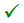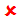# Recap chart : available models list

## Présentation

All the models presented in this chapter are presented in the following chart with the information of their availability in Flux and the material manager.

B(H) for soft materials Flux Material manager
Linear Linear isotropicLinear anisotropic
Linear complex Linear isotropic complex
Linear anisotropic complex
Non linear Isotropic analytic saturation (arctg, 2 coef)
Anisotropic analytic saturation (arctg, 2 coef)
Isotropic analytic saturation + knee adjustment (arctg, 3 coef)
Anisotropic analytic saturation + knee adjustment (arctg, 3 coef)
Isotropic spline saturation
Anisotropic spline saturation
Rayleigh isotropic: parabola + straight line
Hysteresis Jiles-Atherton vector hysteresisB(H) for magnets Flux Material manager
Linear

Linear magnet described by the Br moduleLinear magnet described by the Br module, with Br linear function of the temperatureLinear magnet described by cartesian vector BrLinear magnet described by cylindrical vector Br
Linear magnet described by spherical vector Br
Non linear Nonlinear magnet described by Hc and Br moduleB(H, T) Flux Material manager
Linear Linear isotropic * exponential function of TLinear isotropic, tabulated function of T
Nonlinear Isotropic analytic saturation * exponential function of T
Isotropic analytic saturation + knee adjustment * exponential function of T
Isotropic analytic saturation, tabulated function of T
D(E) Flux Material manager
Linear Linear isotropicLinear anisotropic
Linear complex Linear isotropic with losses (tg δ )
Linear anisotropic with losses (tg δ )
Non linear Isotropic splineJ(E) Flux Material manager
InsulatorIsotropic resistivity
Anisotropic resistivity
Isotropic splineIsotropic superconductivityJ(E, T) Flux Material manager
Isotropic resistivity, linear function of TAnisotropic resistivity, linear functions of T
Isotropic resistivity, exponential function of T
Anisotropic resistivity, exponential functions of T
Isotropic resistivity, tabulated function of T
k(T) Flux Material manager
Isotropic conductivity, constant (independent from T)Anisotropic conductivity, constant (independent from T)
Isotropic conductivity, linear function of T
Anisotropic conductivity, linear functions of T
Isotropic conductivity, exponential function of T
Anisotropic conductivity, exponential functions of T
Isotropic conductivity, tabulated function of T
ρCp(T) Flux Material manager
Specific heat, constant (independent from T)Specific heat, linear function of T
Specific heat, exponential function of T
Specific heat, Gaussian function of T + constant
Specific heat, Gaussian function of T + exponential
Specific heat, tabulated function of T